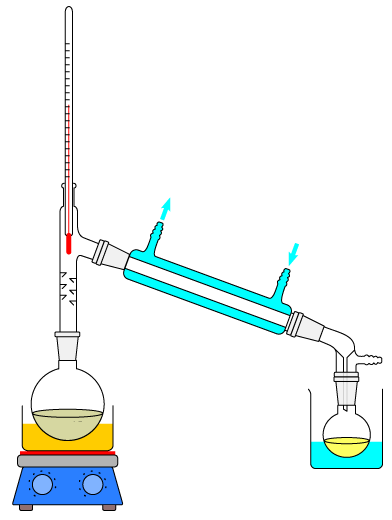Chemistry µGCSE:

Separations

10 quick questions - for GCSE and iGCSE

10 minutes maximum! (can you do it in 5?)

Q1-3 refer to the following particle diagrams. Different atoms are represented by orange and blue circles.Select the diagram which represents ……..

1. .. a mixture of gases.
2. .. a gaseous compound.
3. .. chlorine gas (Cl2)

4. Which of the following is correct about diagrams X and Y?Diagram X represents.. Diagram Y represents.. A two elements a mixture B two elements a compound C two compounds a mixture D two compounds a compound
5. Which type of substance can be separated by filtration?
• A.   an element
• B.   a compound
• C.   a mixture
• D.   a molecule
6. This apparatus can be used to obtain pure water from sea water.What is the name of the process shown?

• A.   filtration
• B.   evaporation
• C.   crystallisation
• D.   distillation

Q7-9. Select the most suitable technique to obtain the first named substance from each of the following mixtures:

7. Chalk from a mixture of chalk and water.
8. Kerosene from crude oil.
9. Copper(II)sulfate from copper(II)sulfate solution.

10. A student investigated the colour in three different flowers X, Y and Z.

He set up the apparatus as shown:An error made by the student was he ..

• A.   put a lid on the beaker
• B.   drew the start line in ink
• C.   put the start line above the water level
• D.   put all three spots on the same piece of chromatography paper